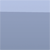# Class Resources

### Font Error

There is a problem viewing many of the following files through Preview in Mac OS Snow Leopard. Some of the symbols (left parentheses, for example) are missing. I don't know if the problem is with Adobe's encoding or with Snow Leopard's decoding. The files do look fine in previous generations of the OS, and they continue to look fine in Adobe Acrobat.

### For Pre-Calculus

Transformations of functions. A page illustrating the different elementary transformations of functions: translations, scalings, and reflections.

Trigonometric Identities. A formula sheet.

### For Calculus

Delta-epsilon proof. An example

Derivative formula sheet. The formulas for the inverse trigonometric functions, the hyperbolic functions, the inverse hyperbolic functions, the logarithmic and the exponential functions.

Rolle's Theorem. An example.

The Riemann sum. An example.

Methods of integration. Several pages summarizing the different integration techniques (substitution, integration by parts, trigonometric integrals, trigonometric substitution, and partial fractions).

Disks and shells. Illustration of the disk and shell methods to calculate volumes of revolution.

Common polar curves. Graphs of circles, cardioids, limacons, lemniscates, and petal curves.

Conic sections. The ellipse, hyperbola and parabola. Illustrated and labeled.

Taylor series. A formula sheet for the Taylor series of the exponential, sine, cosine, and natural logarithm functions, as well as the geometric series.

### For upper-level classes

Cosets. Visualizing cosets in the symmetric group S3 and the alternating group A4.

Hilbert's axioms. Two pages listing Hilbert's axioms, with simple illustrations.

### Processing sketches

These sketches were produced with Processing, a Java based programming environment.

Azulejo Graphs. Pictures of an integer lattice under various combinations of linear transformations.

The Group of Units. This illustrates the orders of the elements in U(n), up to n=50.

Polar Graphs. An example that shows the relationship between a polar graph and its Cartesian counterpart.

Fixed points of Mobius maps. A Mobius map is a complex map which has the form f(z)=(az+b)/(cz+d) with ad-bc≠0. The applet illustrates the flow lines and the fixed points of a Mobius map.

Mobius DFS. This shows the complex interplay between two Mobius transformations by plotting the fixed points of many of the different possible compositions of those two maps. As described in Indra's Pearls by Mumford and Wright.